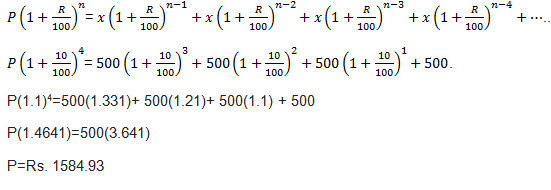Question 1: Four equal installments, each of Rs. 500, were paid at the end of the year on a sum borrowed at 10% compounded annually. Find the sum (correct up to 2 decimal place).

a. Rs. 1584
b. Rs. 1456
c. Rs. 1390
d. Rs. 1595

Option a

Rate= 10%

Installment = Rs.500

Principal=?

Putting these values in the formula:Question 2: A sum of Rs. 240 is to be repaid in 3 equal annual installments. If rate =20% compounded annually, then the value of each installment will be? (rounded off to a whole number)
a. Rs. 120
b. Rs. 140
c. Rs. 114
d. Rs. 116

Option c

Let the value of installment be p

Equating the amounts

240x(1.2)3=p+1.20p +1.44p

p= Rs. 114

Question 3: Calculate the amount of equal installment, which will discharge a debt of Rs. 4240 due in 4 years at 4% p.a. of simple interest.
a. Rs. 1200
b. Rs. 1000
c. Rs. 1104
d. Rs. 1160

Option bQuestion 4: A sum of Rs. 12300 is borrowed at 5% pa compound interest and paid back in 2 equal annual installments. What is the amount of each installment?
a. Rs. 6615
b. Rs. 6800
c. Rs. 7300
d. Rs. 5600

Option a

Let the value of installment be x

Equating the amounts

6150*(1.05)2=x+1.05x

x= Rs. 6615

Question 5: A TV set is sold for Rs.880 cash or for Rs.400 cash down payment together with Rs.488 to be paid after one month. Find the rate of interest charged in the installment scheme.
a. Rs. 12%
b. Rs. 10%
c. Rs. 20%
d. Rs. 11%

Option c

Principal for the next month = 880 – 400 = 480

Amount paid after next month = 488

Therefore interest charged at Rs.480 = 480×1×R/12×100 which is equal to Rs.8

(As interest = principal – amount = 488-480) Therefore, Rate of interest (R) = 20% per annum

Practice Tests

Get Posts Like This Sent to your Email
Updates for Free Live sessions and offers are sent on mail. Don't worry: we do not send too many emails..:)
Get Posts Like This Sent to your Email
Updates for Free Live sessions and offers are sent on mail. Don't worry: we do not send too many emails..:)